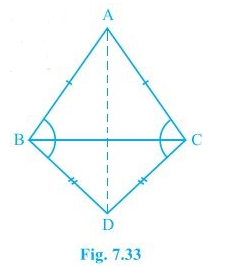# ABC and DBC are two isosceles triangles on the same base BC (see Fig.) . Show that ABD = ACD.#### Solution

Given

AB=AC,BD=DC

To Prove

∠ABD = ∠ACD

Proof

From ∆ABD,

∴∠1=∠2 [angle opposite to equal sides are equal] 

In ∆BDC,

BD=DC

∴∠3=∠4 [angle opposite to equal sides are equal] 

∠1+∠2= ∠2+∠4

∠ABD=∠ACD

Hence Proved